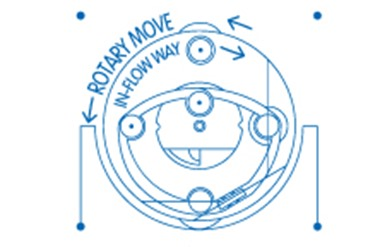Search
Mar 19, 2022Science and Technology
Physically Can Apply Positive/in favor Newton's III Third Law of Motion as a Cycle System in a Motor Engine Device

3rd Thermodynamic Law Applicate in a Power-Plant Device. The Reaction at From of the Action. New Torque Power. It’s a simple Plus Formula of the Interaction of the Two Powers: 1/Action, + 2/Reaction = Retrodynamic. / For every action there is an equal and opposite reaction. 2 Forces in 1 System.

RETRODYNAMIC Effect - Dextrogiro => vs <= Levogiro Phenomena Effect. / Rotor/RPM VS InFlow/Way. 2dn Thermodynamic Law Applicated in a Power-Plant Device. The Reaction at From of the Action. New Torque Power. Its a simple Pluse Formula of the Interaction of the Two Powers: 1/Accion, + 2/Reaction = Retrodynamic.

"Changing rotation inside a mass makes it possible to change its inertial properties. It is the equation for a jet motion without rejection of any mass.” Albert Einstein.

The formula of the Retrodinamic effect is a simple mathematical summation. What is Dextrogiro plus Levogiro, gives the result of the sum, of what is the total, which is the retrodynamic effect. But keeps doing Exponential.

The logic of creation of an inertial propulsion system is thus: Any motion is rotation ---- Rotation of a matter generates a space-time Torsion ---Torsion of space - time is described by Ricci torsion --- Ricci torsion is an inertial field-----the rest mass of any object is determined by its inertial field---- operating by fields and forces of inertia inside of mass we can create inertial propulsion system which moves according to the equation [m (t) dv/dt =-vdm/dt].

Newton's Third Law of Motion: III. For every action there is an equal and opposite reaction.

It is the sum of both (two) Forces - one to other / Are two Forces in one system.

Retrodynamic Effect, are two Forces Actives in one system.
It's like when the Star Trek Enterprise Spaceship Accelerates into the depth of space but spinning 2Two Opposites Forces (Like the Ying-Yang Concept) on a Central Axis in a Rotary Manner Way.

http://stateoftheartnovelinflowtech.blogspot.com

https://www.behance.net/gearturbina61aShare
Carlos Barrera*New Math Symbol: I deal with the Requirements of a new mathematical symbol, in order to explain the Retrodynamic Phenomenon that occurs in an invention that I am developing. It is called the "Retrodynamic Effect" and it is created by having a situation of an axis turning in the opposite direction in a rotating system and in this way a great force is created. And there is currently no mathematical symbol that can explain it.
Mar 19, 2022
Carlos BarreraIt's because I'm talking about something that you can't even think is physically possible.
And in my invention ·1-Gearturbine: I present it already in a system that works in a mechanical Efficiency simple way.
You can appreciate it simply by following the direction of the internal flow, opposite in favor of the blade/Planetary Gear.
And contrary to the Rotary Move. Thats Retrodynamic.
Just Follow the the Dynamic Motion at the cycle System.
It's not only concern of one force/Action. Are two forces:
Action +Plus Reaction = Retrodynamic
Mar 19, 2022